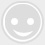# 【忆恋分享】项链的长度多少寸才合适

-回复 -浏览1
3 =16 =40.64 cm
1
5 =18 =45.72 cm
1
6 =20 =50.80 cm
1
8 =22 =55.88 cm
2
0 =24 =60.96 cm
2
2 =26 =66.64 cm

------这里是忆恋珠宝。